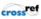Please wait a minute...
 Chin. Phys. B, 2020, Vol. 29(3): 030201    DOI: 10.1088/1674-1056/ab6964
 GENERAL Next

# Lump and interaction solutions to the (3+1)-dimensional Burgers equation

Jian Liu(刘健)1, Jian-Wen Wu(吴剑文)2
1 Department of Mathematics and Physics, Quzhou University, Quzhou 324000, China;
2 Department of Physics, Zhejiang Normal University, Jinhua 321004, China
Abstract  The (3+1)-dimensional Burgers equation, which describes nonlinear waves in turbulence and the interface dynamics, is considered. Two types of semi-rational solutions, namely, the lump-kink solution and the lump-two kinks solution, are constructed from the quadratic function ansatz. Some interesting features of interactions between lumps and other solitons are revealed analytically and shown graphically, such as fusion and fission processes.
Keywords:  (3+1)-dimensional Burgers equation      lump solution      interaction wave solution      bilinear form
Received:  06 November 2019      Revised:  27 December 2019      Published:  05 March 2020
 PACS: 02.30.Jr (Partial differential equations) 02.70.Wz (Symbolic computation (computer algebra)) 02.60.Cb (Numerical simulation; solution of equations)
Fund: Project supported by the National Natural Science Foundation of China (Grant Nos. 11501323, 11701323, and 11605102).
Corresponding Authors:  Jian Liu     E-mail:  jian.liu_math@qzc.edu.cn

#### Cite this article:

Jian Liu(刘健), Jian-Wen Wu(吴剑文) Lump and interaction solutions to the (3+1)-dimensional Burgers equation 2020 Chin. Phys. B 29 030201

  Dauxois T and Peyrard M 2006 Physics of Solitons (Cambridge: Cambridge Univ. Press)  Manakov S V, Zakhorov V E and Bordag L A 1977 Phys. Lett. 63 205Leblond H and Manna M 2007 Phys. Rev. Lett. 99 064102Bergshoeff E and Townsend P K 1999 J. High Energy Phys. 9905 021  Falcon E, Laroche C and Fauve S 2002 Phys. Rev. Lett. 89 204501Ma W X 2015 Phys. Lett. A 379 1975Ma W X and Zhou Y 2017 J. Differential Equations 264 2633  Zhang H Q and Ma W X 2018 Nonlinear Dyn.87 2305  Guo F and Lin J 2019 Nonlinear Dyn. 96 1233Ren B, Ma W X and Yu J 2019 Comput. Math. Appl.77 2086  Yang Y Q, Wang X and Cheng X P 2018 Wave Motion 77 1Hao X Z, Liu Y P, Li Z B and Ma W X 2019 Comput. Math. Appl. 77 724Yang Y Q, Yan Z Y and Malomed B A 2015 Chaos 25 103112Wang X B, Tian S F, Qin C Y and Zhang T T 2017 Appl. Math. Lett. 68 40Ren B, Ma W X and Yu J 2019 Nonlinear Dyn. 96 717Tang Y N, Tao S Q, Zhou M L and Guan Q 2017 Nonlinear Dyn. 89 429Liu J G and He Y 2018 Nonlinear Dyn. 92 1103Ma W X, Yong X L and Zhang H Q 2018 Comput. Math. Appl. 75 289Lou S Y and Lin J 2018 Chin. Phys. Lett. 35 050202Kofane T C, Fokou M, Mohamadou A and Yomba E 2017 Eur. Phys. J. Plus 132 465An H L, Feng D and Zhu H X 2019 Nonlinear Dyn. 98 1275Li W T, Zhang Z, Yang X Y and Li B 2019 Int. J. Mod. Phys. B 22 1950255  Dai C Q and Wang Y Y 2009 Phys. Lett. A 373 181Yin J P and Lou S Y 2003 Chin. Phys. Lett. 20 1448Dai C Q and Yu F B 2014 Wave Motion 51 52Tang X Y and Lou S Y 2003 Chin. Phys. Lett. 20 335Wang H 2018 Appl. Math. Lett. 85 27Wang S, Tang X Y and Lou S Y 2004 Chaos Solitons Fract. 21 231Lump, lumpoff and predictable rogue wave solutions to a dimensionally reduced Hirota bilinear equation Haifeng Wang(王海峰), Yufeng Zhang(张玉峰). Chin. Phys. B, 2020, 29(4): 040501.  Localized characteristics of lump and interaction solutions to two extended Jimbo-Miwa equations Yu-Hang Yin(尹宇航), Si-Jia Chen(陈思佳), and Xing Lü(吕兴). Chin. Phys. B, 2020, 29(12): 120502.  Dynamics of three nonisospectral nonlinear Schrödinger equations Abdselam Silem, Cheng Zhang(张成), Da-Jun Zhang(张大军). Chin. Phys. B, 2019, 28(2): 020202.  Lump-type solutions of a generalized Kadomtsev-Petviashvili equation in (3+1)-dimensions Xue-Ping Cheng(程雪苹), Wen-Xiu Ma(马文秀), Yun-Qing Yang(杨云青). Chin. Phys. B, 2019, 28(10): 100203.  A more general form of lump solution, lumpoff, and instanton/rogue wave solutions of a reduced (3+1)-dimensional nonlinear evolution equation Panfeng Zheng(郑攀峰), Man Jia(贾曼). Chin. Phys. B, 2018, 27(12): 120201.  Interactions among special embed-solitons for the (3+1)-dimensional Burgers equation Zhang Wen-Ting, Dai Chao-Qing, Chen Wei-Lu. Chin. Phys. B, 2013, 22(4): 040509.  Grammian and Pfaffian solutions as well as Pfaffianization for a (3+1)-dimensional generalized shallow water equation Tang Ya-Ning, Ma Wen-Xiu, Xu Wei. Chin. Phys. B, 2012, 21(7): 070212.
 No Suggested Reading articles found!